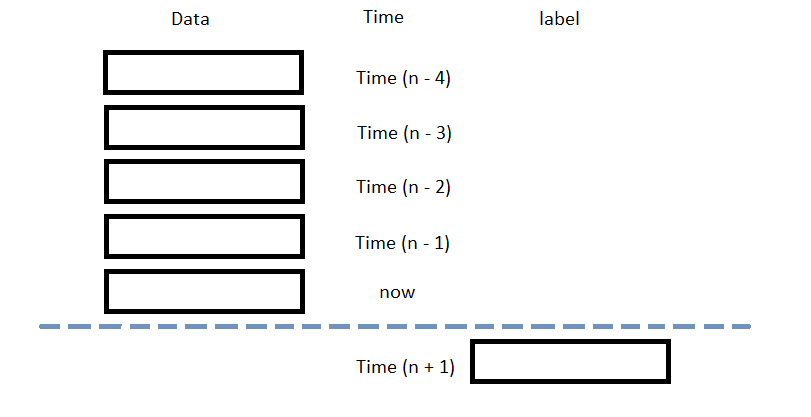# Popular Libraries

## Keras

### Introduction

This page explains how to build, train, deploy and store Keras models.

### Import Libraries

Import the keras libraries.

from AlgorithmImports import *
from tensorflow.keras.layers import Dense, Flatten
from tensorflow.keras.optimizers import Adam

### Create Subscriptions

In the Initialize method, subscribe to some data so you can train the keras model and make predictions.

self.symbol = self.AddEquity("SPY", Resolution.Daily).Symbol

### Build Models

In this example, build a neural-network regression model that uses the following features and labels:

Data CategoryDescription
FeaturesDaily percent change of the open, high, low, close, and volume of the SPY over the last 5 days
LabelsDaily percent return of the SPY over the next day

The following image shows the time difference between the features and labels:Follow the below steps to build the model:

1. In the Initialize method, create a Sequential object with several layers.
2. self.model = Sequential([Dense(10, input_shape=(5,5), activation='relu'),
Dense(10, activation='relu'),
Flatten(),
Dense(1)])

Set the input_shape of the first layer to (5, 5) because each sample contains the percent change of 5 factors (percent change of the open, high, low, close, and volume) over the previous 5 days. Call the Flatten constructor because the input is 2-dimensional but the output is just a single value.

3. Call the compile method with a loss function, an optimizer, and a list of metrics to monitor.
4. self.model.compile(loss='mse',
metrics=['mae', 'mse'])

### Train Models

You can train the model at the beginning of your algorithm and you can periodically re-train it as the algorithm executes.

#### Warm Up Training Data

You need historical data to initially train the model at the start of your algorithm. To get the initial training data, in the Initialize method, make a history request.

training_length = 252*2
self.training_data.Add(trade_bar)

#### Define a Training Method

To train the model, define a method that fits the model with the training data.

def get_features_and_labels(self, n_steps=5):
daily_pct_change = training_df.pct_change().dropna()

features = []
labels = []
for i in range(len(daily_pct_change)-n_steps):
features.append(daily_pct_change.iloc[i:i+n_steps].values)
labels.append(daily_pct_change['close'].iloc[i+n_steps])
features = np.array(features)
labels = np.array(labels)

return features, labels

def my_training_method(self):
features, labels = self.get_features_and_labels()
self.model.fit(features, labels, epochs=5)

#### Set Training Schedule

To train the model at the beginning of your algorithm, in the Initialize method, call the Train method.

self.Train(self.my_training_method)

To periodically re-train the model as your algorithm executes, in the Initialize method, call the Train method as a Scheduled Event.

# Train the model every Sunday at 8:00 AM
self.Train(self.DateRules.Every(DayOfWeek.Sunday), self.TimeRules.At(8, 0), self.my_training_method)

#### Update Training Data

To update the training data as the algorithm executes, in the OnData method, add the current TradeBar to the RollingWindow that holds the training data.

def OnData(self, slice: Slice) -> None:
if self.symbol in slice.Bars:
self.training_data.Add(slice.Bars[self.symbol])

### Predict Labels

To predict the labels of new data, in the OnData method, get the most recent set of features and then call the predict method.

features, _ = self.get_features_and_labels()
features = features[-1].reshape(1, 5, 5)
prediction = float(self.model.predict(features)[-1])

You can use the label prediction to place orders.

if prediction > 0:
self.SetHoldings(self.symbol, 1)
elif prediction < 0:
self.SetHoldings(self.symbol, -1)

### Save Models

Follow these steps to save keras models into the Object Store:

1. Set the key name you want to store the model under in the Object Store.
2. model_key = "model"
3. Call the GetFilePath method with the key.
4. file_name = self.ObjectStore.GetFilePath(model_key)

This method returns the file path where the model will be stored.

5. Call the save method with the file path.
6. self.model.save(file_name)

You can load and trade with pre-trained keras models that you saved in the Object Store. To load a keras model from the Object Store, in the Initialize method, get the file path to the saved model and then call the load_model method.

def Initialize(self) -> None:
if self.ObjectStore.ContainsKey(model_key):
file_name = self.ObjectStore.GetFilePath(model_key)
self.model = load_model(file_name)

The ContainsKey method returns a boolean that represents if the model_key is in the Object Store. If the Object Store does not contain the model_key, save the model using the model_key before you proceed.

### Clone Example Algorithm

You can also see our Videos. You can also get in touch with us via Discord.